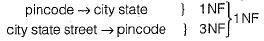Courses

# Functional Dependencies And Normalization (Advance Level)- 1

## 15 Questions MCQ Test Question Bank for GATE Computer Science Engineering | Functional Dependencies And Normalization (Advance Level)- 1

Description
This mock test of Functional Dependencies And Normalization (Advance Level)- 1 for Computer Science Engineering (CSE) helps you for every Computer Science Engineering (CSE) entrance exam. This contains 15 Multiple Choice Questions for Computer Science Engineering (CSE) Functional Dependencies And Normalization (Advance Level)- 1 (mcq) to study with solutions a complete question bank. The solved questions answers in this Functional Dependencies And Normalization (Advance Level)- 1 quiz give you a good mix of easy questions and tough questions. Computer Science Engineering (CSE) students definitely take this Functional Dependencies And Normalization (Advance Level)- 1 exercise for a better result in the exam. You can find other Functional Dependencies And Normalization (Advance Level)- 1 extra questions, long questions & short questions for Computer Science Engineering (CSE) on EduRev as well by searching above.
QUESTION: 1

### A functional dependency of the form X → Y is trivial if

Solution:

A function dependency of from X → Y is trivial when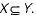QUESTION: 2

### In an entity relationship, y is the dominant entity and x is a subordinate entity. Which of the following is/are incorrect?

Solution:

1. y is dominant entity.

2. x is subordinate entity..
Since, y is dominant entity. So does not depend on any other and x is subordinate. So x is existence dependent on y and deletion of x does not effect y, So, x is detected, so y is incorrect.

QUESTION: 3

### Relations produced from an E-R model will always be in

Solution:

Relations produced from E-R model generally in 1-NF form.

QUESTION: 4

Let R be a relation. Which of the following com m ents about the relation R are correct?
1. R will necessarily have a composite key if R is in BCNF but not in 4NF.

2. If R is in 3NF and if every key of R is simple , then R is in BCNF.

3. If R is in BCNF and if R has at least one

4. If R is in 3NF and if its every key is simple, then R is in 5NF.

Solution:
QUESTION: 5

Consider the relation:
Employee (Emp-No, Emp-name, salary, project- no, due-date)
(Assuming an 1-1 relationship between project and employees)
Project-no is functionally dependent on

Solution:

The relation EMPLOYEE has one to one relation b/w project and employee i.e. each employee has being assigned a project and each project is associated with only one employee, hence project- number is functionally dependent on Emp-no.

QUESTION: 6

Let R ( a, b, c) and S (d, e, f) be two relations in which d is the foreign key of S that refers to the primary key of R. Consider the following four operations.
1. Insert into R
2. Insert into S
3. Delete from R
4. Delete from S

Which of the following is true about the referential integrity constraint about?

Solution:

R (a, b, d, c) and S (e,f)
1. Insertion into R does not create any violation,
2. Insertion into S create violation.
3. Deletion in S does not create any violation.
4. Deletion in R can create violation.

QUESTION: 7

Consider the following set of functional dependencies on the scheme (A, B, C).
A —> BC, B —> C, A —> B ,A B —> C
The canonical cover for this set is

Solution:

For the relation (A, B, C)
A —> BC, B —> C, A —> B, AB —> C
The canonical cover is A —> BC and B —> C which is functionally determining all the FD’s represented in the relation and is minimally satisfying all the non-trivial relationships.

QUESTION: 8

Which of the following statement is false?

Solution:

BCNF relation may or may not preserve dependency also any relation cannot be necessarily decomposed into BCNF relation,

QUESTION: 9

R(A, B, C, D) with FD’s
F(A →B, A →C, A → D) and  R1 (A, B, D) with FD’s
F1(A —> B, A → D) and R2(B, C) with FD's
F2 = { }
​Then find which statements is true:

Solution: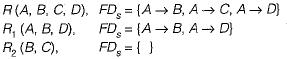Clearly we can see that B is the common attribute and B is not candidate key of any of the decomposed relations. Hence, dependency is not preserved.

QUESTION: 10

R = (A, B, C, D, E, F)
R1 - (A, B)
R2 = (C,D,E)
R3 = (A, C, F)
F= {A→B; C → DE ; AC → F;}
​Does the above FD’s has lossless join property?

Solution:

R = {A, B, C, D, E, F}
F = {A → B , C → DE, AC → F)
R1 = ( A B )
R2 =.{C,D,E}
R3 = {A, C, F}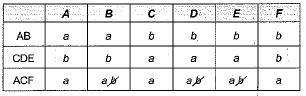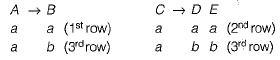Convert b to a in 3rd row convert b to a in 3rd row. Since 3rd row contains all ‘a’s hence the relation has lossless join property.

QUESTION: 11

Which of the following list represents the MVDs (Mean Value Dependencies), satisfied by a relation R(A, B, C) that has the following tuples: (a1, b1, c1),(a1, b1, c2), (a2, b1, c1), (a2, b1,c3).

Solution: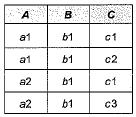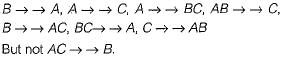QUESTION: 12

From the following instance of a relation schema R(A, B, C), we can conclude that: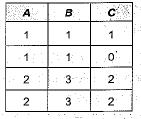Solution:

From the given instance we can determine that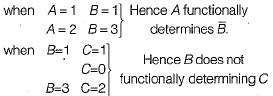QUESTION: 13

Consider the following declaration:
F: Set of functional dependencies
R: Relation of functional dependencies . R : (A, B, C, D, E)
F: (A —> BC: CD —> E; B —> D; E —> A)
Then candidate keys for R is ___________

Solution:

R = (A, B, C, D, E)
F = (A →BC, C D → E, D,B → D, E→A)
A+ = ABCDE so A is the candidate key.
A can be replaced by E
So, E also a candidate key.
Scan be replaced by CD.
Hence, (A, E, C, D) are the candidate keys.

QUESTION: 14

A given relation is known to be in third normal form. Select the statement which can be inferred from this

Solution:

A relation is in 3NF if for X →A
(i) X is a super key or candidate key
(ii) A is a prime attribute.
Hence, for A to be non-key attribute (non-prime attribute)
X must be satisfying (i)

QUESTION: 15

A relation Empdtl is defined with attribute empcode (unique), name, street, city, state and pincode. For any pincode, there is only one city and state. Also, for any given street, city and state, there is just one pincode. in normalization terms, Empdtl is a relation in

Solution:

Empdt 1 (empcode, name, street, city, state, pincode) given for any pincode there is only one city and state
i.e.  pincode→ city state.
Also for any given street, city and state there is only one pincode.
i.e. city state street pincode. key for the relation is thus {empcode name pincode street} prime attributes = {empcode, name, pincode, street}
Now the FD’s